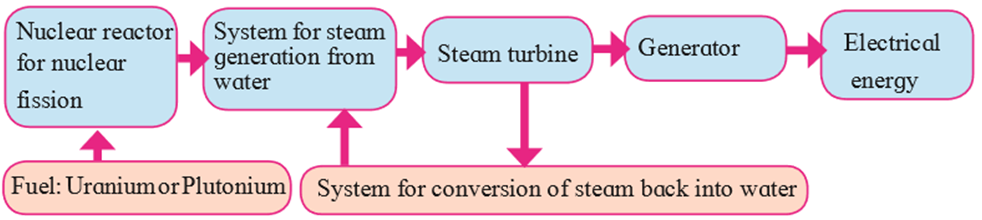SSC BOARD PAPERS IMPORTANT TOPICS COVERED FOR BOARD EXAM 2024

### Which type/types of power generation involve maximum number of steps of energy conversion? In which power generation is the number minimum?

Question 4.

Which type/types of power generation involve maximum number of steps of energy conversion? In which power generation is the number minimum?

NUCLEAR POWER PLANT involves maximum number of steps in the process of transformation.
1. First, nuclear energy is released by fission of nuclei of atoms like uranium or plutonium.
2. The nuclear energy released is used to generate steam (thermal energy) of high temperature and high pressure.
3. The thermal energy is transformed into kinetic energy in steam.
4. The kinetic energy in steam, is then converted into kinetic energy in the turbine.
5. The turbine rotates hence generating electrical energy.ELECTRIC GENERATOR USING WIND involves the minimum steps in the process of transformation.
1. The kinetic energy in the wind is used in wind power plants
2. This kinetic energy is converted to kinetic energy in the wind-turbine. Wind-turbine is a machine which converts the kinetic energy of the wind to electrical energy.
3. The turbine rotates hence generating electrical energy.